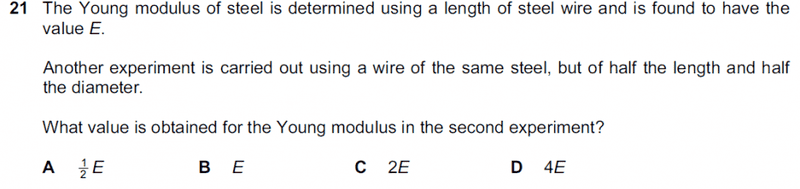# Finding Young's Modulus

## Homework Statement## Homework Equations

E= (F/A) x (L/ΔL)

## The Attempt at a Solution

I know that since the material is the same, the Young modulus should be the same. However, when I try to find the ratio of the second wire to the first, I get the answer C. For the first wire, E= 4FL / d2Δl, since A = d2/4.
For the second wire, the value of E I obtain is F x ½L / (d2/16) x Δl , which is twice the first value. I can't see what's wrong with my working. Can someone point it out?